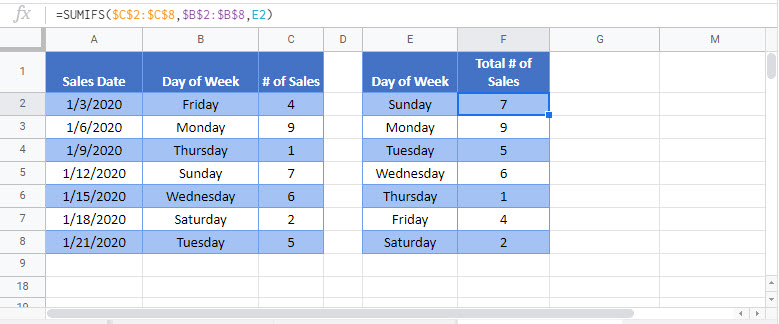# sum if by day of week – Excel & Google Sheets

This tutorial will demonstrate how to use the SUMIFS Function to sum data corresponding to specific days of the week in Excel and Google Sheets.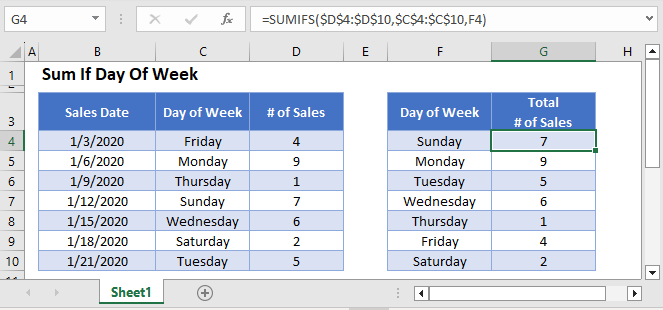## Sum If by Day of Week – Text Values

First, we will demonstrate how to sum data corresponding to specific Day of Week names.

We can use the SUMIFS Function, along with a helper column containing the TEXT Function to sum all Sales that take place on a specific Day of Week:

``=SUMIFS(D3:D9,C3:C9,F3)``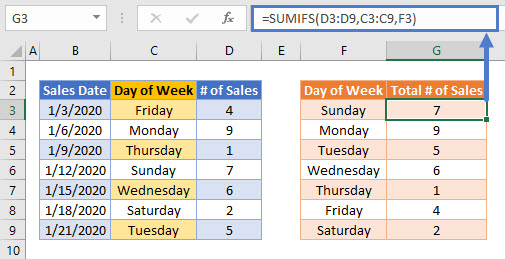In the above example, we use the TEXT Function to extract the name of the Day of Week from the Sales Date:

``=TEXT(B3,"dddd")``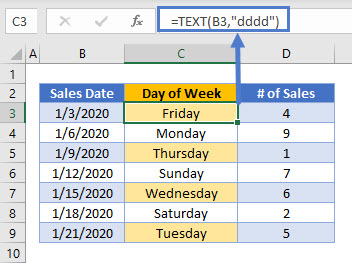The SUMIFS Function then uses this helper column to produce the summary table:

``=SUMIFS(D3:D9,C3:C9,F3)``## Sum If by Day of Week – Using WEEKDAY

Alternatively, weekday numbers can be used. To do this, the helper column in the source data needs to use the WEEKDAY Function:

``=WEEKDAY(B3)``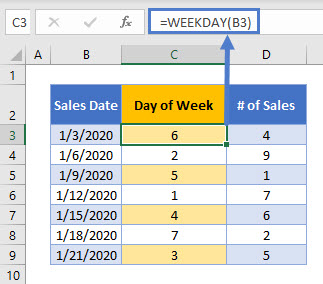Using the WEEKDAY numerical value instead of the weekday name may provide you with more flexible options for later data visualization tasks and summary functions.

## Sum If by Day of Week – Without Helper Column

The above example summed data by weekday using a helper column. To avoid the need for a helper column, we can use the SUMPRODUCT Function:

``=SUMPRODUCT(--(WEEKDAY(B3:B9)=E3),C3:C9)``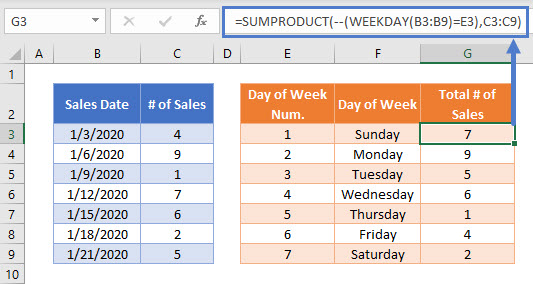Here, the SUMPRODUCT Function performs a complicated “sum if” calculation. Let’s walk through the above example.

This is our final formula:

``=SUMPRODUCT(--(WEEKDAY(B3:B9)=E3),C3:C9)``

First, the SUMPRODUCT Function evaluates the weekday number of each of the Sales Dates and also lists the array of values from Number of Sales column:

``=SUMPRODUCT(--({6; 2; 5; 1; 4; 7; 3}=1),{4; 9; 1; 7; 6; 2; 5})``

Using the logical test (=1), weekday numbers of 1 are changed to TRUE, all others are FALSE:

``=SUMPRODUCT(--({FALSE; FALSE; FALSE; TRUE; FALSE; FALSE; FALSE }),{4; 9; 1; 7; 6; 2; 5})``

Next the double dashes (–) convert the TRUE and FALSE values into 1s and 0s:

``=SUMPRODUCT({0; 0; 0; 1; 0; 0; 0 },{4; 9; 1; 7; 6; 2; 5})``

The SUMPRODUCT Function then multiplies each pair of entries in the arrays to produce an array of Number of Sales on Sales Dates that match Day of Week 1:

``=SUMPRODUCT({0; 0; 0; 7; 0; 0; 0})``

Finally, the numbers in the array are summed:

``=7``

More details about using Boolean statements and the “–” command in a SUMPRODUCT Function can be found here

## Locking Cell References

To make our formulas easier to read, we’ve shown the formulas without locked cell references:

``=SUMPRODUCT(--(WEEKDAY(B3:B9)=E3),C3:C9)``

But these formulas will not work properly when copy and pasted elsewhere in your file. Instead, you should use locked cell references like this:

``=SUMPRODUCT(--(WEEKDAY(\$B\$3:\$B\$9)=E3),\$C\$3:\$C\$9)``